# PARTNERS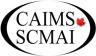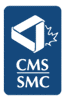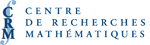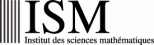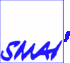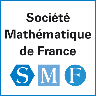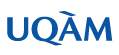Plenary Speakers
[PDF]

YVES ANDRÉ, CNRS-ENS, Paris
Slope filtrations and their metamorphoses
[PDF]

Many "slope filtrations" have been introduced in algebraic geometry, asymptotic analysis, ramification theory and p-adic theories. We shall outline a unified treatment of their common features and roles, and survey how new ties between different domains have been woven by dint of deep correspondences between some slope filtrations.

OLIVIER BIQUARD, Université Paris 6
Conformal geometries and Einstein metrics
[PDF]

In 1986 Fefferman and Graham proposed a new approach to conformal geometry, by considering a correspondence between conformal geometry in dimension n+1 and Einstein metrics in dimension n. In 1997 physicists (Maldacena, Witten) discovered that this is a part of a physical correspondence called AdS/CFT correspondence. From this new viewpoint emerged several important progress in conformal geometry or its variants like CR geometry (the geometry of the boundaries of complex domains).

I will explain the general philosophy of the AdS/CFT correspondence and discuss recent constructions and results.

LUC DEVROYE, McGill University
Random trees
[PDF]

Random trees appear in many contexts. We make an attempt at unifying various models encountered in computer science, discrete mathematics, combinatorics and biology. Parameters of interest to us include the height, the profile and the shape of these trees. Various recent developments in this respect will be reviewed.

ANDREW GRANVILLE, Université de Montréal
Pretentiousness in analytic number theory
[PDF]

Inspired by the "rough classification" ideas from additive combinatorics, Soundararajan and I have recently introduced the notion of pretentiousness into analytic number theory. Besides giving a more accessible description of the ideas behind the proofs of several well-known difficult results of analytic number theory, it has allowed us to strengthen several results, like the Polya-Vinogradov inequality, the prime number theorem, etc. In this talk we will introduce these ideas and gave some flavour of these developments.

ALICE GUIONNET, ENS Lyon, CNRS, 46 allée d'Italie, 69364 Lyon Cedex 07, France
Topological Expansions and Applications
[PDF]

We will review recent developments in random matrix theory related with the enumeration of maps, which are connected graphs embedded into a surface.

In the seventies, Brézin, Itzykson, Parisi and Zuber, following an idea of 't Hooft, showed that Gaussian matrix integrals expand formally as generating functions for the enumeration of maps sorted by their genus. This follows from Gaussian calculus (or Feynman diagrams). We shall prove that these `topological' expansions are, under appropriate assumptions, also asymptotic expansion as the size of the matrices goes to infinity. This in particular justifies the use of Gaussian matrix integrals in physics to enumerate these graphs.

We will show that such `topological' expansions generalize to other matrices such as unitary matrices following the Haar measure (even though Gaussian calculus does not hold anymore). We will finally discuss some applications in combinatorics and operator algebras.

RICK KENYON, Brown University, Providence, RI 02912, USA
Limits of Dimer models
[PDF]

The dimer model has a very simple definition: counting weighted perfect matchings on a graph. (A perfect matching of a graph is a set of edges covering all the vertices exactly once.) The dimer model on planar graphs has many connections with other parts of modern mathematics, for example random matrices, Young tableaux, SLE, random surfaces, and many others.

This talk will survey some of these connections, showing in particular how by taking various limits one can use the dimer model to model crystal surfaces, the Gaussian free field, SLEk for k=2,4,6,8, Dyson Brownian motion, annihilating/coalescing Brownian motions, and a number of other processes.

GÉRARD LAUMON, CNRS and Université Paris-Sud
Hitchin Fibration and Fundamental Lemma
[PDF]

The Fundamental Lemma, which has been conjectured by Robert Langlands and Diana Shelstad, is a set of combinatorial identities between p-adic orbital integrals. It plays a key role in the stabilization of the elliptic part of the Arthur-Selberg trace formula. A generalization, due to James Arthur, the weighted Fundamental Lemma, is required to stabilize the non-elliptic terms.

The Langlands-Shelstad Fundamental Lemma has been proved in general by Ngô Bao Châu as a consequence of his cohomological study of the elliptic part of the Hitchin fibration. With Pierre-Henri Chaudouard we have extended Ngô Bao Châu's arguments in order to obtain the weighted Fundamental Lemma.

In this talk I would like to present these recent advances.

Le Lemme Fondamental, conjecturé par Robert Langlands et Diana Shelstad, est un ensemble d'identités combinatoires entre intégrales orbitales p-adiques. Il joue un rôle central dans la stabilisation de la formule des traces d'Arthur-Selberg. Une généralisation, due à James Arthur, le Lemme Fondamental pondéré, est nécessaire pour stabiliser les termes non elliptiques.

Le Lemme Fondamental de Langlands-Shelstad a été prouvé en général par Ngô Bao Châu comme conséquence de son étude cohomologique de la partie elliptique de la fibration de Hitchin. Avec Pierre-Henri Chaudouard nous avons étendu les arguments de Ngô Bao Châu pour obtenir le Lemme Fondamental pondéré.

Dans cet exposé, j'aimerais présenter ces développements récents.

ERIC SERE, CEREMADE, Université Paris-Dauphine, Place du Maréchal de Lattre de Tassigny, 75775 Paris Cedex 16, France
Variational problems in Relativistic Quantum Chemistry
[PDF]

This talk is based on a series of joint works with J. Dolbeault, M. J. Esteban, C. Hainzl and M. Lewin, on stationary solutions of linear and nonlinear equations from Relativistic Quantum Chemistry, involving the Dirac operator. The solutions are found as critical points of an energy functional under normalization constraints. Contrary to the Laplacian appearing in the equations of nonrelativistic Quantum Mechanics, the Dirac operator has a negative continuous spectrum which is not bounded from below. As a consequence, the energy functional is strongly indefinite, i.e., all its critical points have an infinite Morse index. The associated Euler-Lagrange equations are linear or nonlinear eigenvalue problems with eigenvalues lying in a spectral gap. Moreover, since we work in the space domain R3, the Palais-Smale condition is not satisfied. For these reasons, the problems discussed here pose a challenge in the Calculus of Variations. The existence proofs involve sophisticated tools from nonlinear analysis and have required new variational methods which are now applied to other problems.

I will focus on the problematic definition of the "ground state" of atoms and molecules in Relativistic Quantum Chemistry, keeping in mind computational issues which are essential in this field. In nonrelativistic Quantum Mechanics, the "ground state" is simply a minimizer of the energy under normalization constraints. But in the Dirac-Fock model, which is commonly used in numerical relativistic calculations, the energy is not bounded below. Therefore the definition, existence proof and numerical computation of the "ground state" require much more care.

JEAN-PIERRE SERRE, Collège de France
Le groupe de Cremona
[PDF]

NICOLE TOMCZAK-JAEGERMANN, University of Alberta
Random embeddings and approximation and other high-dimensional geometric phenomena
[PDF]

This talk will illustrate a geometric and probabilistic approach of Asymptotic Geometric Analysis to several high-dimensional phenomena described by a large class of random matrices. Those include Gaussian and +1/-1 matrices, more generally, subgaussian matrices, and also matrices determined by subsets of bounded orthogonal systems. We shall consider random embeddings of normed spaces (notably, of the Euclidean space), random approximation and random projections, used for example in compressed sensing, and some properties of combinatorial flavor of random 0/1 polytops. These phenomena are intimately connected to probabilistic inequalities for singular numbers of a wide class of random matrices.

NIZAR TOUZI, CREST-Paris
Probabillistic numerical methods for fully-nonlinear PDEs
[PDF]

The theory of backward stochastic differential equations allows to extend the Feynman-Kac representation result to the case of quasilinear PDEs. By analogy with the linear case, this representation opened the door for probabilistic numerical methods for these equations. This result was further extended to fully nonlinear PDEs by means of the notion of second order backward stochastic differential equations. We provide convergence results for the numerical schemes suggested by this probabilistic representation, together with bounds on the rate of convergence.

JIANHONG WU, MITACS Center for Disease Modeling, York University
Disease outbreaks and outbreaks of disease modeling
[PDF]

Shortly after the 2002-03 Severe Acute Respiratory Syndrome (SARS) outbreak, a Canadian team on modeling communicable diseases was established, and an adventure of interdisciplinary research involving close interaction and collaboration between mathematical/statistical modelers, medical scientists, and public health policy makers was started.

This talk provides a brief review of the history of this team, its collective efforts and long-term goal in modeling emerging/reemerging communicable diseases of critical importance to Canada and the international community. It also presents some progress in modeling pandemic influenza and in analysis of such issues as antiviral treatment and drug resistance.

The team has been asked on various occasions to talk about what it has achieved using mathematical modeling. The team has also been asked to report the impact of its work on public health policy development, and to document the influence of its research and networking activities on the training of the next generation of modelers, and on encouraging health care people to believe in modeling. This talk not only provides partial answers to the above questions, but also raises more questions to challenge modelers.